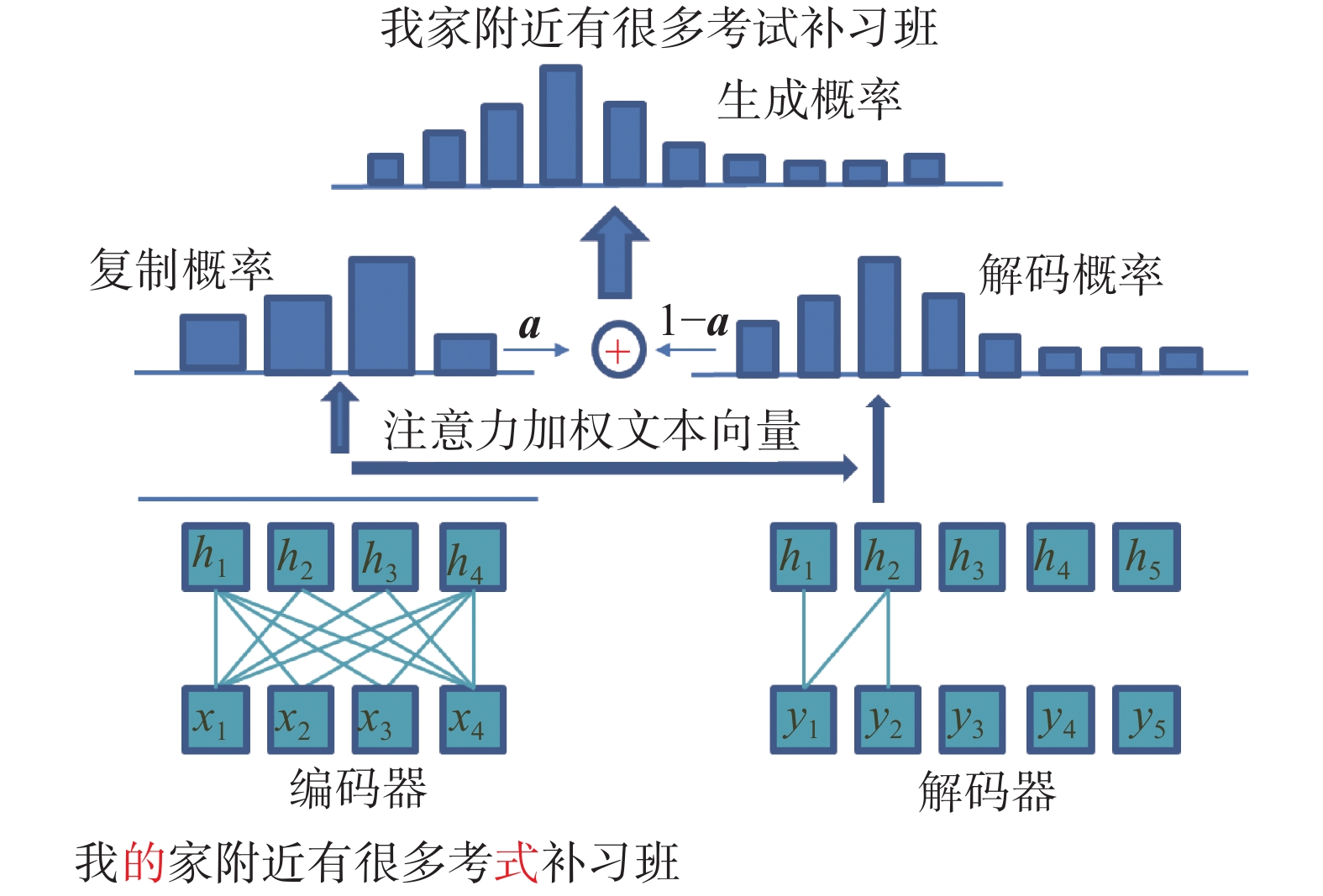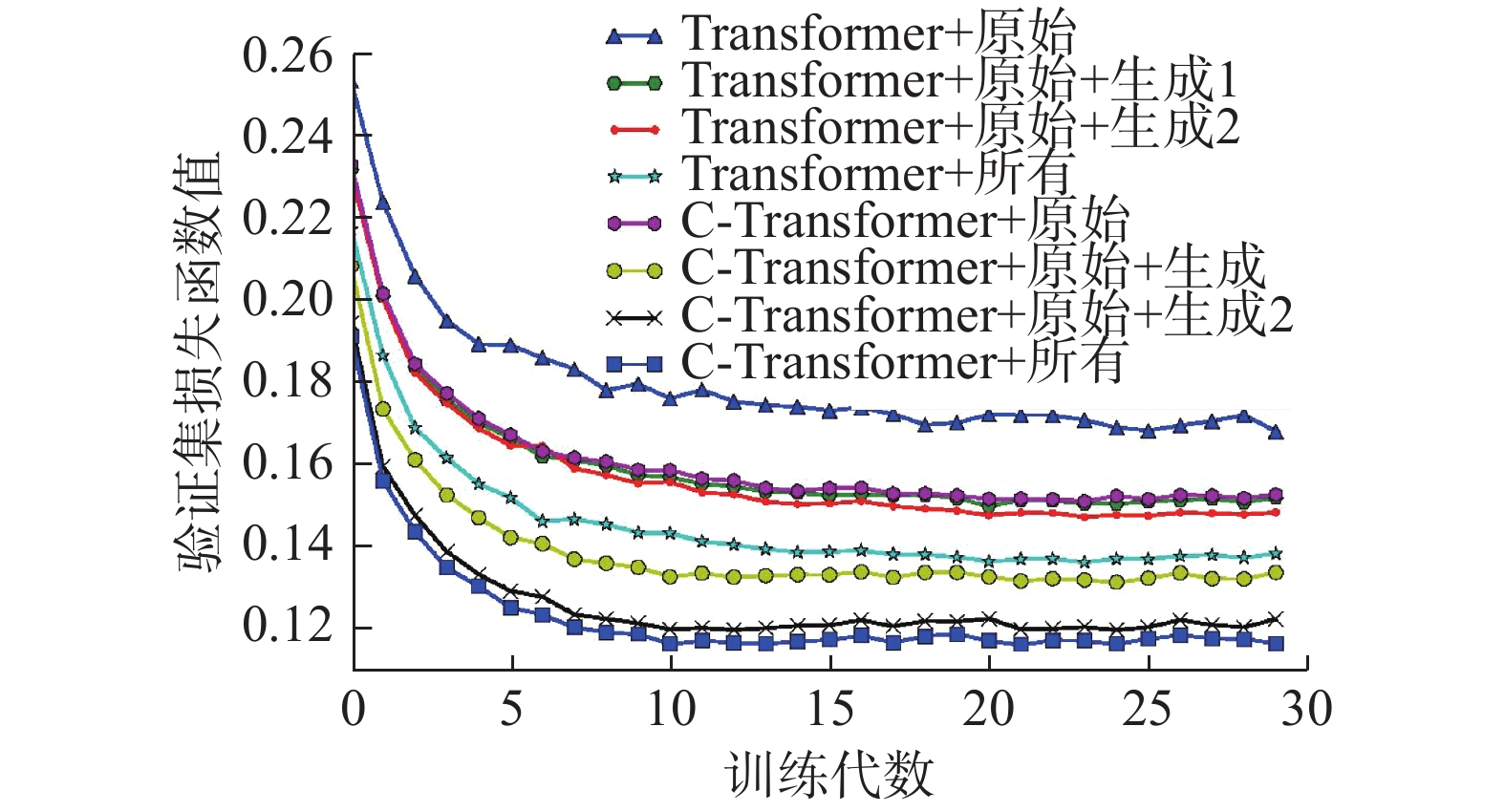﻿ 基于数据增广和复制的中文语法错误纠正方法
«上一篇文章快速检索 高级检索

 智能系统学报2020, Vol. 15Issue (1): 99-106  DOI: 10.11992/tis.2020010140

### 引用本文WANG Quanbin, TAN Ying. Chinese grammatical error correction method based on data augmentation and copy mechanism[J]. CAAI Transactions on Intelligent Systems, 2020, 15(1): 99-106. DOI: 10.11992/tis.202001014.### 文章历史

Chinese grammatical error correction method based on data augmentation and copy mechanism
WANG Quanbin , TAN YingSchool of Electronics Engineering and Computer Science, Peking University, Beijing 100871, China
Abstract: Chinese is a widely used language. However, due to its natural difference between Indo-European languages, Chinese learners tend to make various grammatical errors. This article proposes an automatic grammar correction method for those who will make errors like typos or improper words order. First, we built the C-Transformer model that adopts copy mechanism in the self-attention model to translate wrong text sequence to the correct one. Second, based on the public data set, a pure sequence to sequence method is utilized to generate wrong text corresponding to the correct one, and an error text filter is designed based on fluency, semantic, and syntactic measurements. Finally, since Chinese words are pictographic, based on the collected homographs and homophones dictionaries, some error samples are artificially constructed to expand training data. The experimental results show that our method can well correct typos, improper word order, missing, redundancy and other errors, and achieved the state-of-the-art performance on the standard test set of Chinese text grammatical error correction.
Key words: self-attention mechanism    copy mechanism    sequence to sequence learning    Chinese    grammatical error correction    neural networks    text generation    fluency

1 文本语法错误纠正表 1 训练样本示例 Tab.1 Some examples in the training data
2 相关工作

3 C-Transformer模型Download: 图 1 C-Transformer模型结构 Fig. 1 The model architecture of C-Transformer
3.1 序列到序列学习

 $\widehat {{\theta }} = \mathop {{\rm{argmax}}}\limits_{{\theta }} \sum\limits_{({{x}},{{y}}) \in { T}} {\log p({{y}}|{{x}};{{\theta }})}$ (1)

 $p({{y}}|{{x}};\widehat {{\theta }}) = \prod\limits_{i = 1}^L {p({y_i}|{{x}},{{{y}}{ < i}};\widehat {{\theta }})}$ (2)
3.2 Transformer模型

1) 缩放点积注意力(scaled dot-product attention)缩放点积注意力机制的核心如式(3)所示：

 ${\rm{Attention}}({{Q,K,V}}) = {\rm{softmax}}\Bigg(\frac{{{{Q}}{{{K}}^{\rm{T}}}}}{{\sqrt {{d_k}} }}\Bigg){{V}}$ (3)

 $\begin{split} &{\rm{MultiHead}}({{Q,K,V}})\! =\! {\rm{Concat}}({\rm{hea}}{{\rm{d}}_1}, {\rm{hea}}{{\rm{d}}_2},\cdots ,{\rm{hea}}{{\rm{d}}_h}){{{W}}^O}\\ &{\rm{hea}}{{\rm{d}}_i} = {\rm{Attention}}({{QW}}_i^Q{{,KW}}_i^K{{,VW}}_i^V) \end{split} \!\!\!\!\!\!\!\!\!\!\!\!\!\!\!\!\!\!\!\!\!\!\!\!$ (4)

3) 编码(Positional Encoding)

 $\begin{array}{l} {\rm{PE}}({\rm{pos}},2i) = {\rm{sin}}({\rm{pos}}/{10\;000^{2i/d}}) \\ {\rm{PE}}({\rm{pos}},2i + 1) = {\rm{cos}}({\rm{pos}}/{10\;000^{2i/d}}) \\ \end{array}$ (5)

3.3 复制机制

 ${p_t}(w) = (1 - a_t^{}) \times p_t^{\rm{*}}(w) + a_t^{}\times p_t'(w)$ (6)

 $a_t^{} = {\rm{sigmoid(}}{{{W}}^{\rm{T}}}\sum {({{({q}_t^{\rm{T}}{{K}})}^{\rm{T}}} \cdot {{V}})} {\rm{)}}$ (7)

$p_t'(w)$ $t$ 时刻解码的词是从原文复制的概率，从公式8计算得到：

 $p_t'(w) = {\rm{softmax}}({q}_t^{\rm{T}}{{K}})$ (8)

4 数据增广 4.1 数据集表 2 实验数据统计信息 Tab.2 The statistical information of the data

4.2 正确到错误逆序生成

4.3 词表映射生成表 3 混淆集中的同音或同形字示例 Tab.3 Some examples of the confusion set with similar pronunciation or shape

5 实验结果与分析 5.1 实验参数设置Download: 图 2 不同配置下模型验证集损失函数值 Fig. 2 The loss on validation set with different settings
5.2 评价指标

 $\begin{array}{l} P = \dfrac{{\displaystyle\sum\limits_{i = 1}^n {\left| {{e_i} \cap {{\rm{g}}_i}} \right|} }}{{\displaystyle\sum\limits_{i = 1}^n {\left| {{e_i}} \right|} }}\begin{array}{*{20}{c}} {}&{} \end{array}R = \dfrac{{\displaystyle\sum\limits_{i = 1}^n {\left| {{e_i} \cap {{\rm{g}}_i}} \right|} }}{{\displaystyle\sum\limits_{i = 1}^n {\left| {{{\rm{g}}_i}} \right|} }} \\ \begin{array}{*{20}{c}} {\begin{array}{*{20}{c}} {\begin{array}{*{20}{c}} {}&{} \end{array}}&{} \end{array}}&{} \end{array}{F_{0.5}} = \dfrac{{5PR}}{{P + 4R}} \\ \end{array}$ (9)

5.3 实验结果表 4 实验结果对比表 Tab.4 The results of our method on the standard dataset
5.4 分析与讨论表 5 模型修改样本示例 Tab.5 Some examples of the corrected sentences
6 结束语

  VASWANI A, SHAZEER N, PARMAR N, et al. Attention is all you need[C]//Advances in Neural Information Processing Systems. Long Beach, USA, 2017: 5998–6008. (0)  VINYALS O, FORTUNATO M, JAITLY N. Pointer networks[C]//Proceedings of the 28th International Conference on Neural Information Processing Systems. Cambridge, USA, 2015: 2692–2700. (0)  MACDONALD N, FRASE L, GINGRICH P, et al. The writer's workbench: computer aids for text analysis[J]. IEEE transactions on communications, 1982, 30(1): 105-110. DOI:10.1109/TCOM.1982.1095380 (0)  FRANCIS W N, KUCERA H. A standard corpus of present-day edited American English, for use with digital computers[R]. Providence, RI: Department of Linguistics, Brown University, 1979. (0)  MANGU L, BRILL E. Automatic rule acquisition for spelling correction[C]//Proceedings of the Fourteenth International Conference on Machine Learning. San Francisco, USA, 1997: 187–194. (0)  CAHILL A, MADNANI N, TETREAULT J, et al. Robust systems for preposition error correction using Wikipedia revisions[C]//Proceedings of the 2013 Conference of the North American Chapter of the Association for Computational Linguistics: Human Language Technologies. Atlanta, USA, 2013: 507–517. (0)  BROCKETT C, DOLAN W B, GAMON M. Correcting ESL errors using phrasal SMT techniques[C]//Proceedings of the 21st International Conference on Computational Linguistics and the 44th annual meeting of the Association for Computational Linguistics. Stroudsburg, USA, 2006: 249–256. (0)  JUNCZYS-DOWMUNT M, GRUNDKIEWICZ R. Phrase-based machine translation is state-of-the-art for automatic grammatical error correction[C]//Proceedings of the 2016 Conference on Empirical Methods in Natural Language Processing. Austin, Texas, USA, 2016: 1546–1556. (0)  SUTSKEVER I, VINYALS O, LE Q V. Sequence to sequence learning with neural networks[C]//Proceedings of the 27th International Conference on Neural Information Processing Systems. Cambridge, USA, 2014: 3104–3112. (0)  XIE Z, AVATI A, ARIVAZHAGAN N, et al. Neural language correction with character-based attention[J]. arXiv preprint arXiv: 1603.09727, 2016. (0)  WANG Quanbin, TAN Ying. Automatic grammatical error correction based on edit operations information[C]//Proceedings of 25th International Conference on Neural Information Processing. Siem Reap, Cambodia, 2018: 494–505. (0)  GE Tao, WEI Furu, ZHOU Ming. Fluency boost learning and inference for neural grammatical error correction[C]//Proceedings of the 56th Annual Meeting of the Association for Computational Linguistics. Melbourne, Australia, 2018: 1055–1065. (0)  ZHAO Wei, WANG Liang, SHEN Kewei, et al. Improving grammatical error correction via pre-training a copy-augmented architecture with unlabeled data[J]. arXiv preprint arXiv: 1903.00138, 2019. (0)  RAO Gaoqi, ZHANG Baolin, XUN Endong, et al. IJCNLP-2017 Task 1: Chinese grammatical error diagnosis[C]//Proceedings of the IJCNLP 2017. Taipei, China, 2017: 1–8. (0)  WU S H, LIU Chaolin, LEE L H. Chinese spelling check evaluation at SIGHAN Bake-off 2013[C]//Proceedings of the Seventh SIGHAN Workshop on Chinese Language Processing. Nagoya, Japan, 2013: 35–42. (0)  ZHAO Yuanyuan, JIANG Nan, SUN Weiwei, et al. Overview of the NLPCC 2018 shared task: grammatical error correction[C]//Proceedings of 7th CCF International Conference on Natural Language Processing and Chinese Computing. Hohhot, China, 2018: 439–445. (0)  ZHOU Junpei, LI Chen, LIU Hengyou, et al. Chinese grammatical error correction using statistical and neural models[C]//Proceedings of 7th CCF International Conference on Natural Language Processing and Chinese Computing. Hohhot, China, 2018: 117–128. (0)  FU Kai, HUANG Jin, DUAN Yitao. Youdao’s winning solution to the NLPCC-2018 Task 2 challenge: a neural machine translation approach to Chinese grammatical error correction[C]//Proceedings of 7th CCF International Conference on Natural Language Processing and Chinese Computing. Hohhot, China, 2018: 341–350. (0)  HOCHREITER S, SCHMIDHUBER J. Long short-term memory[J]. Neural computation, 1997, 9(8): 1735-1780. DOI:10.1162/neco.1997.9.8.1735 (0)  LECUN Y, BENGIO Y. Convolutional networks for images, speech, and time-series[M]//ARBIB M A. The Handbook of Brain Theory and Neural Networks. Cambridge, USA: MIT Press, 1995: 3361. (0)  REN Honghai, YANG Liner, XUN Endong. A sequence to sequence learning for Chinese grammatical error correction[C]//Proceedings of 7th CCF International Conference on Natural Language Processing and Chinese Computing. Hohhot, China, 2018: 401–410. (0)  BAHDANAU D, CHO K, BENGIO Y. Neural machine translation by jointly learning to align and translate[J]. arXiv preprint arXiv: 1409.0473, 2014. (0)  CHUNG J, GULCEHRE C, CHO K H, et al. Empirical evaluation of gated recurrent neural networks on sequence modeling[J]. arXiv preprint arXiv: 1412.3555, 2014. (0)  SEE A, LIU P J, MANNING C D. Get to the point: summarization with pointer-generator networks[J]. arXiv preprint arXiv: 1704.04368, 2017. (0)  JIA R, LIANG P. Data recombination for neural semantic parsing[J]. arXiv preprint arXiv: 1606.03622, 2016. (0)  LIU CHAOLIN, LAI MINHUA, TIEN K W, et al. Visually and phonologically similar characters in incorrect Chinese words: analyses, identification, and applications[J]. ACM transactions on Asian language information processing, 2011, 10(2): 10. (0)  A project for conversion between traditional and simplified Chinese[EB/OL]. [2019-12-20].https://github.com/BYVoid/OpenCC. (0)